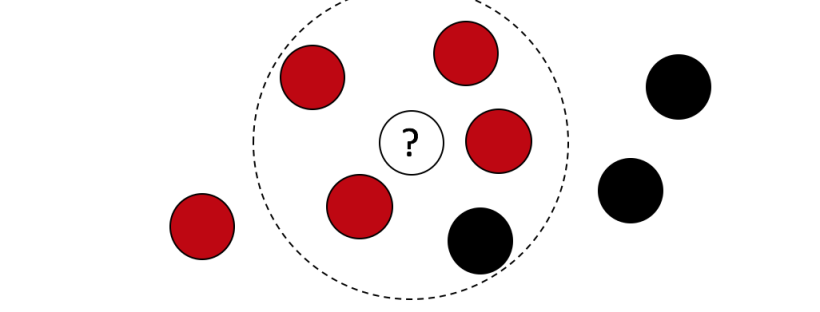# k-nearest Neighbors Regression

In this notebook, you will implement k-nearest neighbors regression. You will:

• Find the k-nearest neighbors of a given query input
• Predict the output for the query input using the k-nearest neighbors
• Choose the best value of k using a validation setIn [None]:

In [None]:

In [None]:

Because the features in this dataset have very different scales (e.g. price is in the hundreds of thousands while the number of bedrooms is in the single digits), it is important to normalize the features

To efficiently compute pairwise distances among data points, we will convert the SFrame into a 2D Numpy array. First import the numpy library and then copy and paste get_numpy_data() from the second notebook of Week 2.

In [None]:

In [None]:

In [None]:

In [None]:

Using all of the numerical inputs listed in feature_list, transform the training, test, and validation SFrames into Numpy arrays:

In [None]:

In computing distances, it is crucial to normalize features. Otherwise, for example, the sqft_living feature (typically on the order of thousands) would exert a much larger influence on distance than the bedrooms feature (typically on the order of ones). We divide each column of the training feature matrix by its 2-norm, so that the transformed column has unit norm.

In [None]:

In [None]:

### Fitting KNN

Find the (error) way to call the features

In [None]:

In [None]:

### Distance functions

The most critical choice in computing nearest neighbors is the distance function that measures the dissimilarity between any pair of observations.

In [None]:

Distance functions are also exposed in the turicreate.distances module. This allows us not only to specify the distance argument for a nearest neighbors model as a distance function (rather than a string), but also to use that function for any other purpose.

In the following snippet we use a nearest neighbors model to find the closest reference points to the first three rows of our dataset, then confirm the results by computing a couple of the distances manually with the Manhattan distance function.

In [None]:

In [None]:

Search methods Another important choice in model creation is the method. The brute_force method computes the distance between a query point and each of the reference points, with a run time linear in the number of reference points. Creating a model with the ball_tree method takes more time, but leads to much faster queries by partitioning the reference data into successively smaller balls and searching only those that are relatively close to the query. The default method is auto which chooses a reasonable method based on both the feature types and the selected distance function. The method parameter is also specified when the model is created. The third row of the model summary confirms our choice to use the ball tree in the next example.

In [None]:

Written on August 16, 2018